# How to VLOOKUP from Different Excel Sheet in Excel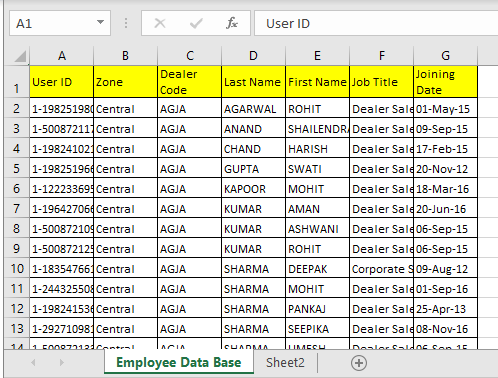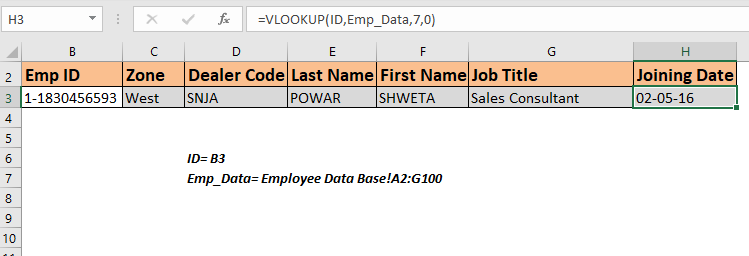In this article, we will learn How to VLOOKUP from Different Excel Sheets in Excel.

How does it matter if the vlookup table is on another sheet ?

To retrieve information from an employee database we can use a VLOOKUP formula. This formula will simply find the unique id and return the information related to that employee ID. If you are new to VLOOKUP functions, this is one of the best VLOOKUP exercises.

VLOOKUP Function with table on different sheet in Excel

Formula Syntax :

 =VLOOKUP(id,database,col,0)

Id: it is the unique id of the employee in the database. We will use it to look up employee information.

Database: It is the table that contains the information of employees. The first column must be the Id.

Col: It is the column number from which you want to retrieve the value.

0 or False: It is used for exact match VLOOKUP.

Or use

Formula Syntax :

 =VLOOKUP(lookup_value, sheetname!table_array, col_index,0)

Here only factor is sheetname! In formula. Just write the correct sheet name in before table_array with ! mark. Excel will VLOOKUP from that given sheet. Let's have a simple example.

Example :

All of these might be confusing to understand. Let's understand how to use the function using an example. Here On sheet1 I have a builder id. On sheet 2 I have the name of those builders. So I just need to fetch names from sheet2 to sheet1 using VLOOKUP.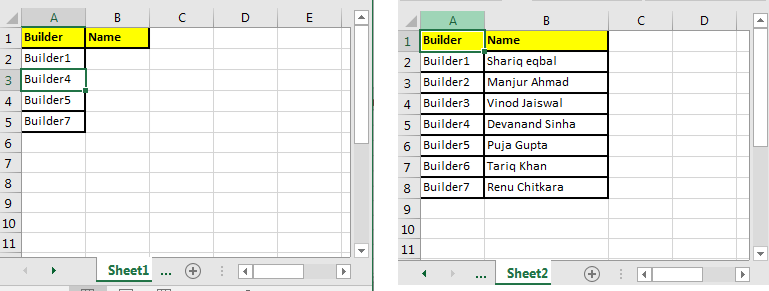Write this formula in cell B2 on sheet1.

 =VLOOKUP(A2,Sheet2!\$A\$2:\$B\$8,2,0)

Note the sheet2 here. If you rename it to sheet3, VLOOKUP will look for data on sheet 3 at range \$A\$2:\$B\$8 (locked the reference to range) if it exists other wise #REF error will be shown.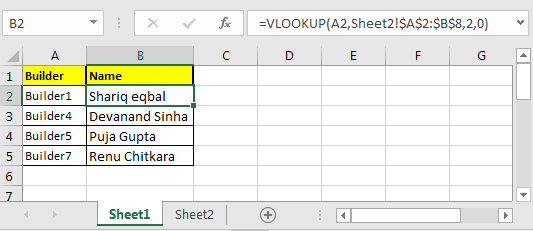Another VLOOKUP Example

Retrieve Employee Information Using VLOOKUP from Employee Table

Here We have a table that contains the details of all the employees in an organization on a separate sheet. The first column contains the ID of these employees. I have named this table as emp_data.Now my search sheet needs to fetch the employee's information whose ID is written in cell B3. I have named B3 as ID.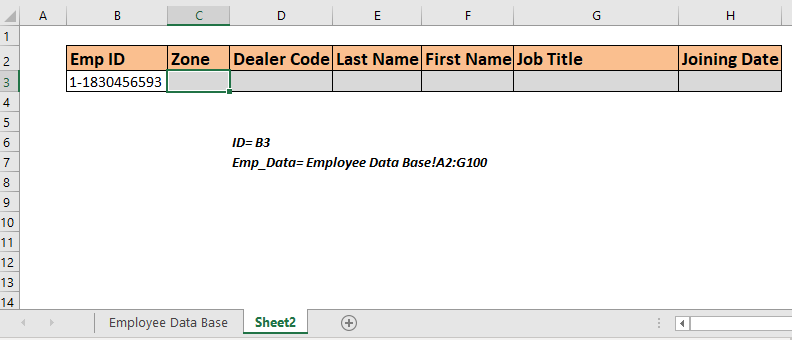For ease of understanding, all the column heading are in exactly the same order as the emp_data  table.

Now write the below formula in cell C3 to retrieve the zone of the employee ID written in B3.

 =VLOOKUP(ID,Emp_Data,2,0)

This will return the zone of the employee Id 1-1830456593 because column number 2 in the database contains the zone of employees.

Copy this formula in the rest of the cells and change the column number in the formula to get all the information of employees.You can see that all the information related to the mentioned ID in Cell B3. Whichever id you write in Cell B3, all the information will be fetched without making any change in the formula.

How does this work?

There's nothing tricky. We are simply using the VLOOKUP function to lookup ID and then fetch the mentioned column. Practice VLOOKUP using such data to understand the VLOOKUP more.

In the above example, we had all the columns organized in the same order but there will be times when you'll have a database that will have hundreds of columns. In such cases, this method of retrieving employee information won't be good. It will be better if the formula can look at the column heading and fetch data from that column from the employee table.

So to retrieve value from the table using column heading we will use a 2-way lookup method or say dynamic column VLOOKUP.

Use this formula in cell C3 and copy in the rest cells. You don't need to change anything in the formula, everything will be fetched from thd emp_data.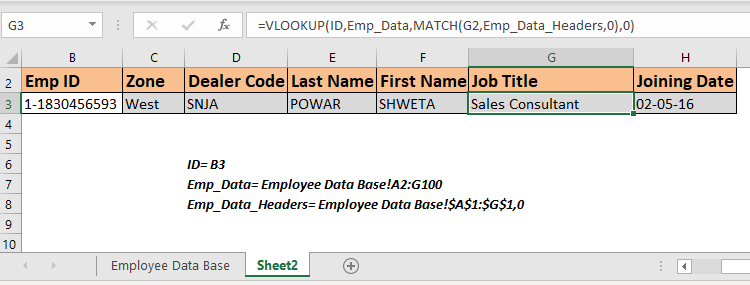This formula simply retrieves all  the information from matched columns. You can jumble the headers in the report, this won't make any difference. Whichever the heading is written in cell above, the respective data contains.

How does this work?

This is simply a dynamic VLOOKUP. You can read about it here. If I'll explain it here, it will become a too large article.

Retrieve Employee ID with Partial Match

It may happen that you don't remember the whole ID of an employee, but you still want to retrieve the information of some ID. In such cases partial match VLOOKUP is the best solution.

For example, If I know that some id contains 2345 but I don't know the whole Id. If I enter this number in Cell C3, the output will be like.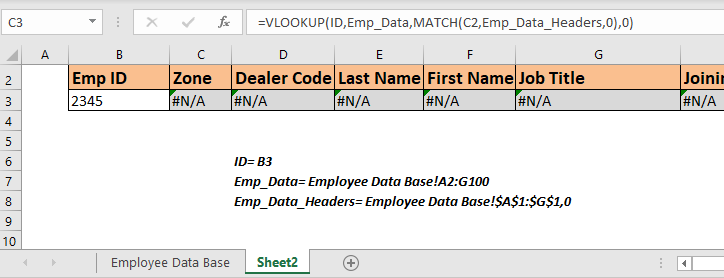We get nothing. Since nothing matches 2345 in the table. Modify the above formula like this.

Copy this in the entire row. And now you have the information of the first employee that contains this number.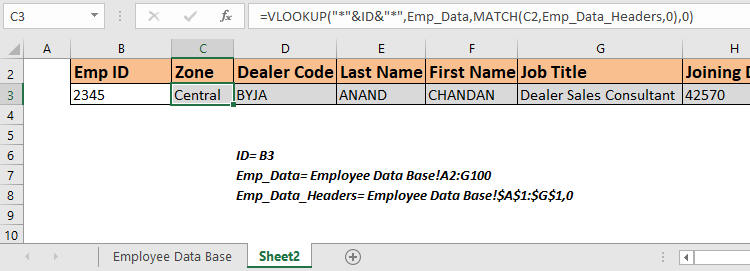Please note that we will get the first ID that contains the matched number in Emp Id column. If any other ID contains the same number, this formula will not retrieve that employee's information.

If you want to get all the employee ids that contain the same number use the formula that lookups all the matched values.

Here are all the observational notes using the formula in Excel
Notes :

1. You can add more rows and columns in the lookup array.
2. Text arguments must be given within quotes ("").
3. The VLOOKUP table must have the lookup_array in the leftmost or the first column.
4. The col index cannot be 1 as it is the lookup array
5. 0 argument is used for the exact match value. Use 1 for the approximate match value.
6. The function returns #N/A error, if look up value is not found in the lookup array. So catch the error, if necessary.

Hope this article about How to VLOOKUP from Different Excel Sheet in Excel is explanatory. Find more articles on calculating values and related Excel formulas here. If you liked our blogs, share it with your friends on Facebook. And also you can follow us on Twitter and Facebook. We would love to hear from you, do let us know how we can improve, complement or innovate our work and make it better for you. Write to us at info@exceltip.com.

Related Articles :

How to Retrieve Latest Price in Excel : It is common to update prices in any business and using the latest prices for any purchase or sales is a must. To retrieve the latest price from a list in Excel we use the LOOKUP function. The LOOKUP function fetches the latest price.

VLOOKUP function to calculate grade in Excel : To calculate grades IF and IFS are not the only functions that you can use. The VLOOKUP is more efficient and dynamic for such conditional calculations.To calculate grades using VLOOKUP we can use this formula.

17 Things About Excel VLOOKUP : VLOOKUP is most commonly used for retrieving matched values but VLOOKUP can do a lot more than this. Here are 17 things about VLOOKUP that you should know to use effectively.

LOOKUP the First Text from a List in Excel : The VLOOKUP function works fine with wildcard characters. We can use this to extract the first text value from a given list in excel. Here is the generic formula.

LOOKUP date with last value in list : To retrieve the date that contains the last value we use the LOOKUP function. This function checks for the cell that contains the last value in a vector and then uses that reference to return the date.

Popular Articles :

How to use the IF Function in Excel : The IF statement in Excel checks the condition and returns a specific value if the condition is TRUE or returns another specific value if FALSE.

How to use the VLOOKUP Function in Excel : This is one of the most used and popular functions of excel that is used to lookup value from different ranges and sheets.

How to use the SUMIF Function in Excel : This is another dashboard essential function. This helps you sum up values on specific conditions.

How to use the COUNTIF Function in Excel : Count values with conditions using this amazing function. You don't need to filter your data to count specific values. Countif function is essential to prepare your dashboard.

Terms and Conditions of use

The applications/code on this site are distributed as is and without warranties or liability. In no event shall the owner of the copyrights, or the authors of the applications/code be liable for any loss of profit, any problems or any damage resulting from the use or evaluation of the applications/code.